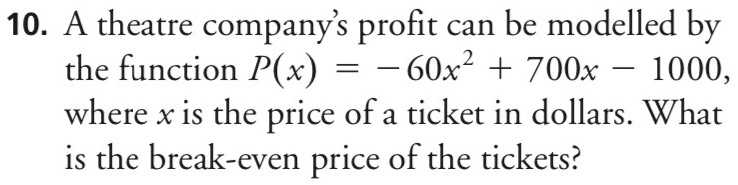### ¿Todavía tienes preguntas de matemáticas?

Pregunte a nuestros tutores expertos
Algebra
PreguntaA theatre company's profit can be modelled by the function $$P ( x ) = - 60 x ^ { 2 } + 700 x - 1000$$ , where $$x$$ is the price of a ticket in dollars. What is the break-even price of the tickets?

$$P(x)= 0,x= 10\text{ or }\frac{5}{3}$$